Next: 3.4 The Source-Filter Model Up: 3. Basic Concepts Previous: 3.2 Additive Analysis and

Subsections

# 3.3 Spectral Envelopes

In this section, I will give a more detailed description of what spectral envelopes  are. A spectral envelope  is a curve in the frequency-amplitude plane, derived from a Fourier magnitude spectrum. It describes one point in time (one window, to be precise). The following properties are desirable for spectral envelopes:

Envelope fit

The curve describes an envelope of the spectrum, i.e. it wraps tightly around the magnitude spectrum, linking the peaks.

Regularity

A certain smoothness or regularity of the curve is required. This means, the spectral envelope must not oscillate too much, but it should give a general idea of the distribution of the signal's energy over frequency. 3.6

We want the curve to be steady (in the mathematical sense of a steady function), i.e. it has no corners (where the first derivative jumps).3.7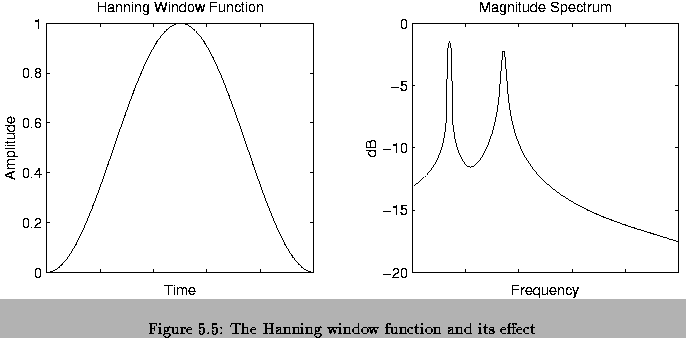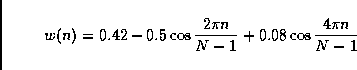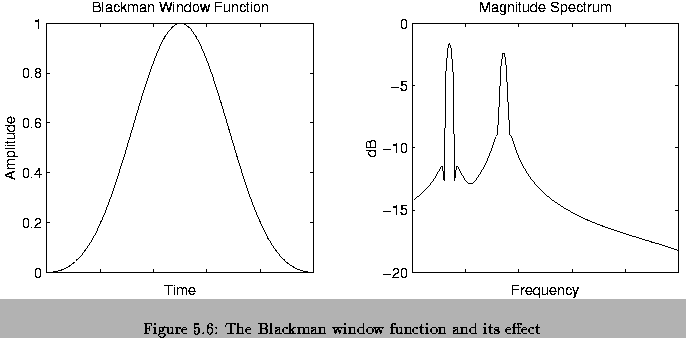From the examples of spectral envelopes in figures 2.20 to 2.22 we see that the characteristics of musical instruments lead to distinctive spectral envelopes. The spectral envelope also reflects the class of a vowel (the phoneme ) in speech, as can be seen in figures 2.23 to 2.25.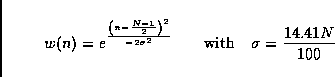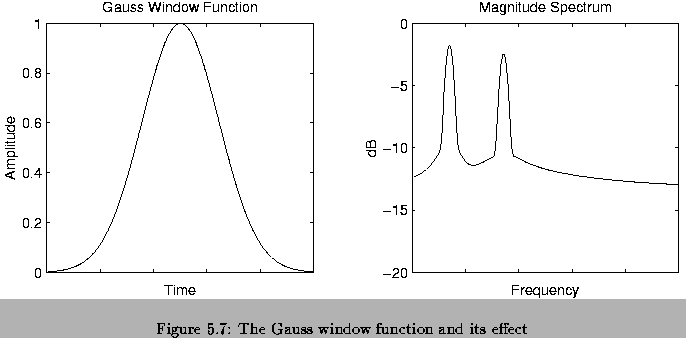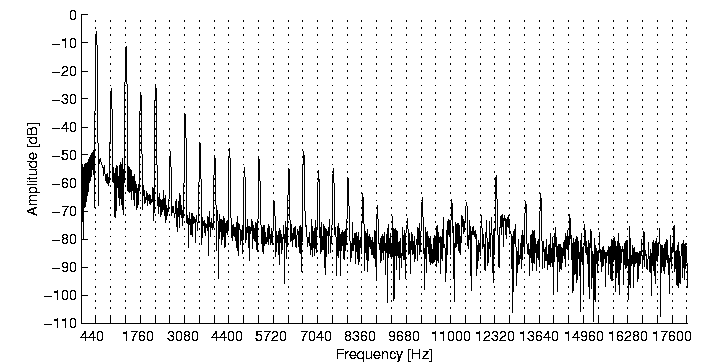## 3.3.1 Spectral Envelope Correction for Transposition

In speech or in the singing voice, the spectral envelope is quite independent of the pitch (see section 2.4 for why this is so). However, if we transpose the vowel in figure 2.23 up by one octave by multiplying the frequencies of all partials by 2 and performing an additive resynthesis, the spectral envelope will necessarily be transposed also. Figure 2.26 shows this effect which sounds quite unnatural (it is sometimes termed the mickey mouse effect ). The unnaturalness comes from the fact that the formants are shifted up one octave, which corresponds to shrinking the vocal tract to half of its length. Obviously, this is not the natural behaviour of the vocal tract.

To avoid this, the spectral envelope has to be kept constant, while the partials slide'' along it to their new values. This means that the amplitude of a transposed partial is no longer determined by the amplitude of the original partial, but by the value of the spectral envelope at the frequency of the transposed partial, as in figure 2.27. This way, only the partials are shifted, but the spectral envelope and thus the formant locations stay the same, making the vowel sound natural.

For an easier comparison, figure 2.28 shows the spectral envelopes of the transposed sound with and without spectral envelope correction, on a frequency grid spaced at 366 Hz intervals, the fundamental frequency of the transposed sound. It can be clearly seen that the partials of both are at the same frequencies, but at different amplitudes, and that one spectral envelope is the stretched version of the other (although the compressed spectral envelope lacks some of the details of the stretched one).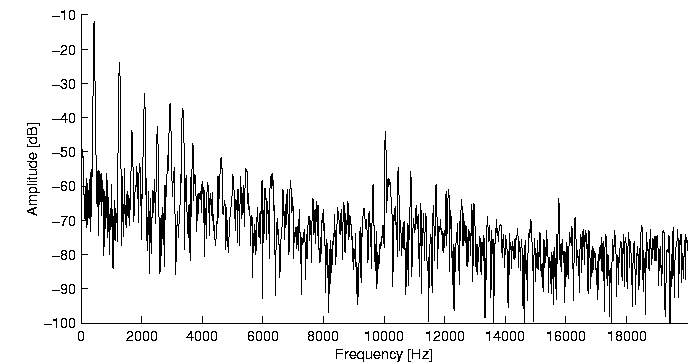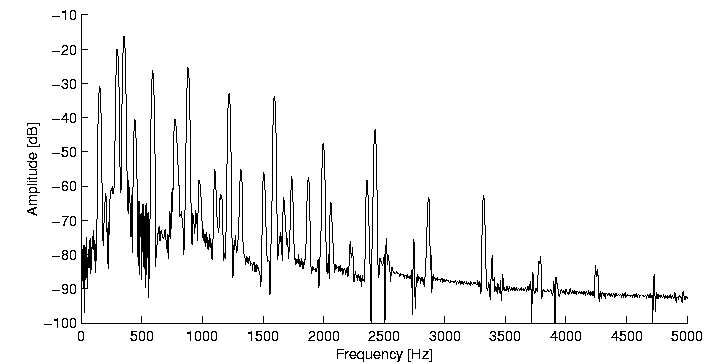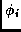## 3.3.2 Vibrato Tracing of Spectral Envelopes

Xavier Rodet remarked that an interesting way to observe the true spectral envelope of the singing voice is to exploit its independence of pitch, making use of the small but fast variation of pitch while singing with a vibrato. In one test recording, during one period of vibrato of c.a. 200 ms, the fundamental frequency f0 oscillates around 149 Hz by a maximum deviation in frequency of b0 = 3.4 Hz. The harmonic partials at k times f0 frequency follow, and oscillate by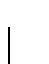. Because the spectral envelope stays fixed, they sweep underneath its curve, tracing small portions of its contour, but nevertheless giving its exact slope.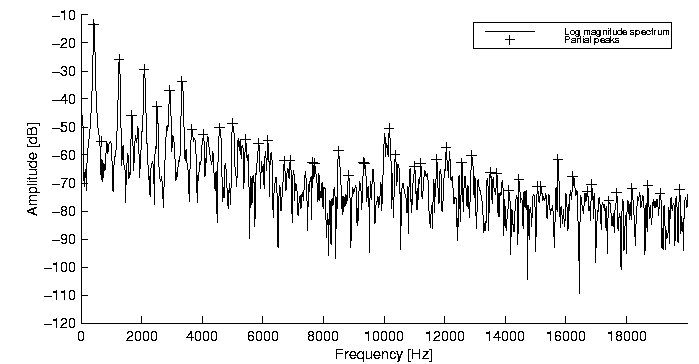tex2html_comment_mark>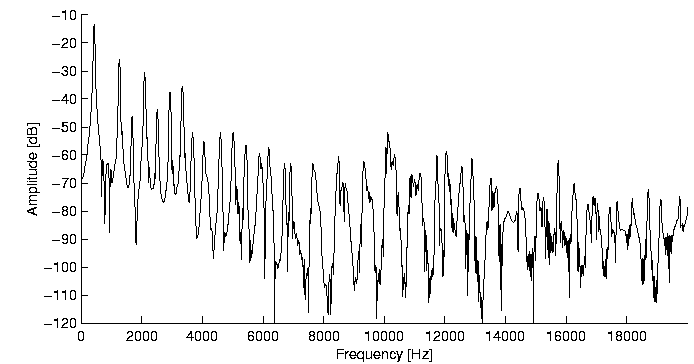tex2html_comment_mark>

Figure 2.29 shows the partials of all the 20 time-frames during one period of a vibrato, collapsed together into one frame. (The data was generated by Nathalie Henrich [Hen98].) In figure 2.30, a close-up of figure 2.29, the traces left by each partial while they follow the oscillation of the fundamental frequency show up as distinct groups of crosses. All in all, at higher frequencies, the amount of irritating factors augments, and more noise is apparent.Next: 3.4 The Source-Filter Model Up: 3. Basic Concepts Previous: 3.2 Additive Analysis and
Diemo Schwarz
1998-09-07